6. Time quantization

Phase diagrams (see for instance QuickStart §4.2) might have unwieldingly many columns representing very short time delays (even less than the current time resolution). Setting a time quantization (an acceptable delay below which two events may be seen as simultaneous, i.e. belong to the same column) helps BP2 to simplify phase diagrams. In many cases this is compulsory for working with complex items in a limited memory space.

The following example demonstrates the quantization process. (Use "-da.tryQuantize") Consider the polymetric expression

{do4 {re4 mi4, fa4 sol4 la4}, si4 do5}
which is interpreted as:

{/6 do4{/6 re4 mi4, /9 fa4 sol4 la4}, /4 si4 do5}
with the phase diagram (if quantization is OFF):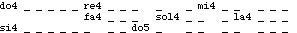Metronome setting mm = 60 produces the following item: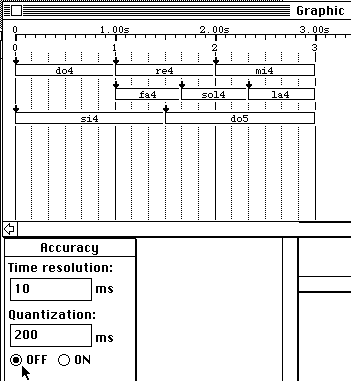Fig.17 No time quantization

The time delay between two consecutive time streaks is 1000 / 6 = 166 milliseconds. This is also the delay between the off-setting of "si4" and the on-setting of "sol4". Let us now use a quantization of 200 ms forcing the interpreter to ignore shorter delays. The new item is shown Fig.18.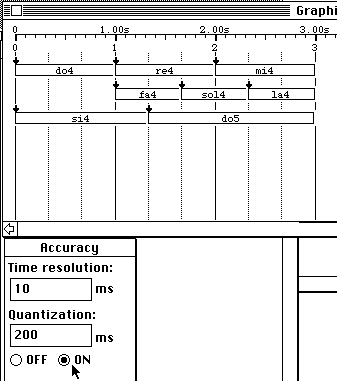Fig.18 Same item with 200 ms quantization

The delay between the off-setting of "si4" and the on-setting of "sol4" is now exactly 200 milliseconds, although it might have become 0 milliseconds in a different context. This amounts to rewriting the item as:

/3{do4_ _ {re4_ _ mi4_ _ ,fa4_ sol4_ la4_},si4_ _ _ do5_ _ _ _}
with the phase diagram: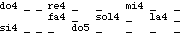Let us take another example. The item shown Fig.14 of QuickStart:

b b <<f>>{5 ,a c b,f - f}a <<chik>> b <<sync>>
was assigned the phase diagram: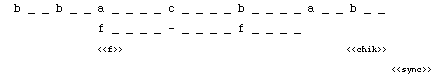Here, for instance, the symbolic duration of "b_ _ b_ _" is six time units. With a mm = 400 metronome setting, the time delay between two time streaks (i.e. two columns of the phase diagram) was 50 milliseconds. If the quantization is set to 60 milliseconds, the phase diagram becomes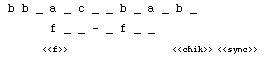which yields the graphic score shown Fig.19.Fig.19 Item with 60 ms quantization

Durations of the first two occurrences of 'b' have become unequal although the total duration of "bb" remained two beats (i.e. 300 milliseconds). In this way, 'a', 'f' and "<<f>>" still have identical on-setting dates. Thus, quantization simplifies the phase diagram as much as possible while maintaining the dates of events within the given range -- here, dates will match exact dates by ±60 milliseconds. In doing so, durations may be adjusted within the acceptable range, but events that were synchronised (on "vertical lines") will remain synchronised .

Setting quantization to any value higher than 50 milliseconds yields the same result. Why? Since the symbolic duration of the first occurrence of 'b' has become one time unit, it cannot become smaller, otherwise sequentiality of the first two occurrences of 'b' would be damaged. The quantization used by BP2 never affects sequentiality of sound-objects. This is a very important feature because any pair of sound-objects may be used to set a process on and off, e.g. NoteOn/NoteOff.

The main advantage of BP2 quantization is an optimisation of computational space and time: it should be used systematically in projects where polymetric structures are likely to yield oversized phase diagrams. In effect, a quantization equal to the time resolution does not change final timings although it is a safeguard against memory overflow.